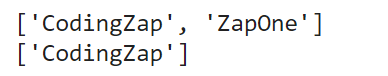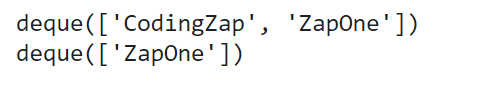# Stack Vs Queue: Which Is Better For Python?

Do you know which one is best in between stack vs queue in Python? Have you ever thought about which needs to be implemented in between the stack vs queue? Let us gain some knowledge about the difference between stack vs queue.

But before we start knowing the best between stack vs queue, we need to first know about them. let us try to figure out the operations from our daily life.

Suppose, you are hungry now. You want to eat some biscuits. So, you asked your mother & she provided the container where she kept the biscuits. The biscuits are kept in a cylindrical manner. This means they are kept one above another. So, now can you take the third biscuit from the container? You will not.

First, you need to prompt out the first biscuit & then the second one. On the way, you will able to eat the third biscuit. Stack works in the same manner. Elements inserted in the stack can able to be removed from one side only.

Now, let us try to assume one scenario for understanding the queue.

Suppose, you are at the railway station. You want to book a ticket for a certain station. Now, at the ticket counter will make a queue there. When you come to the counter, you booked your ticket. Now, can you able to come out from the line where you can enter into? You can’t able to do that.

You need to come out from the line using a point. Then you would not be able to use the same oath to come out from the line. The same thing goes for the queue. The elements that are inserted in the queue, need to be removed from a new path.

So, stack and queue in python are quite clear from the above examples. We intend to find out when to use stack vs queue. Let us first know about the theory of stack and queue in Python briefly.

## What Is Stack? Read Below

The stack is a special data structure element. It is a linear type of data structure. As the elements that are inserted in the stack use a linear path. This means we can easily find them using the stack. Mainly, the stack is implemented with the help of a linked list or array. As both of them are linear in structure, the stack also becomes a linear data structure. There are some operations in the stack. They are known as the Push & Pop operation. Push means to add elements inside of the stack & Pop means to remove the element from the stack.

Stack follows a simple mechanism. This mechanism is known as LIFO. Means Last-In-First-Out. This theory implements that, if any element is inserted inside of the stack first, then the element will remove from the stack at last. As there is only one path to come out from the stack, this theory should be followed. Also, there is a reverse operation. Sometimes it is known as the FILO. It means First-In-Last-Out. FILO & LIFO provide the same theory.## What Is Queue? Read Below

The queue is also a linear data structure. It also follows a linear path while being implemented. Usually, the array or linked list is used for implementing queue operation. Some operations are related to queues. They are Enqueue & Dequeue operations. Enqueue means to add any elements to the queue. And Dequeue means to remove one element from the queue.

The queue also has its mechanism. It is known as the FIFO. It means First-In-First-Out. As there are two ways to the queue. One is to add elements & another is to remove the element. So, any element that is inserted in the queue first, will be removed from the queue first. As the paths are sensitive. The exit path can only be used to remove elements. Also, we can term the theory as LILO. It means, Last-In-Last-Out. They both are the same theory.

After knowing about the stack and queue in Python, it is time to implement those in Python.

### Implementation Of Stack In Python:

Before we start to find out the answer of when to use stack vs queue, it is time to know the implementation of stack in Python. For that purpose, we need to declare one empty list in Python. The list most similarly works as the stack in Python. There using the append() method, we need to push data to the stack. After adding data to it, we need to print the stack.

Now, we will use the pop() operation of the list. This operation will remove the last inserted element from the list. This will follow the theory of the stack. Now, after popping out the element, we need to again print the stack.

Example:

```Stack = [] # Declaring Empty List

Stack.append("CodingZap") # Adding Values To It

Stack.append("ZapOne")

print(Stack) # Printing Total Stack

Stack.pop() # Removing Last Element

print(Stack) # Printing New Stack```

Let us try to find out the output of the above code. It will help to understand the difference between stack vs queue

Output:### Implementation Of Queue In Python:

Now, the queue can also implement using the same approach. But we need to always focus on the theory. In the queue, we need to remove the first element.

Here, we first need to declare an empty list for the queue. Then we enqueue elements there. Now, we need to print the whole queue. After that, we need to remove the first element. We will use the pop() method. but with the help of the index. The zero (0) index will help to point to the first element in the queue.

Example:

```Queue = [] # Declaring Empty Queue

Queue.append("Hello CodingZap") # Adding Values To It

Queue.append("Hello ZapOne")

print(Queue) # Printing Total Queue

Queue.pop(0) # Removing First Element

print(Queue) # Printing New Queue```

Let us try to find out the output of the above code. It will help to understand the difference between stack vs queue

Output:Now, the above method can apply to the queue. But this is not the optimal method. It will take a long time for any large list. Also, this process is quite difficult for deploying any big projects. In the above example, popping data from the beginning is not an easy & quick task for Python. If the list is so long, then there will a problem with the above method.

For that purpose, there is another method. There we need to use the deque() method. This method needs to import. Here, we can able to add elements using the append method. But removing elements will be performed by a special operation. This is known as the popleft() operation. It will remove the first element of the queue without any issues.

Example:

```from collections import deque # Importing Deque

queue = deque() # Declaring Queue Inform Of Deque

queue.append("CodingZap") # Adding Elements To It

queue.append("ZapOne")

print(queue) # Printing The Total Queue

queue.popleft() # Removing The Leftmost Element

print(queue) # Printing The New Queue```

Let us try to find out the output of the above code. It will help to understand the difference between stack vs queue

Output:## When To Use Stack Vs Queue? Get To Know

It is the choice of the user. It should be defined by them only. Stacks can be used in problems where the recursive method is being implemented. On the other hand, the queue can be used at the places where the traditional programming process is going on. Stack uses only one way to enter & remove the elements. On the other hand, the queue needs two different paths for adding & removing the elements.

So, it is advisable to choose the data structure. And implement it in your code. Practice codes as much as possible. It will help to gain knowledge about the subject & the topic.

## Conclusion:

As we saw, it is very important to know the best between stack vs queue.

It will be good to clear the concept of stack and queue in Python first. Then we need to decide when to use stack vs queue.

It is always advisable to clear the basic concept of Python programming language & the data structure concepts for a better understanding of the topic.

Also, looking to gain some more knowledge in the programming world and are curious to know more about binding constraints then you can check out our article

So, hope you have liked this piece of article. Share your thoughts in the comments section and let us know if we can improve more.

Also, if you guys are looking to get help with Python Assignments or Programming then you can use our Python Assignment Help Services.